College Chemistry : Specific Heat Capacity

Example Questions

Example Question #1 : Solutions, States Of Matter, And Thermochemistry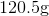block of silver initially at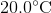absorbs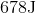of heat. If the specific heat capacity of silver is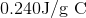, in degrees Celsius, what is the final temperature of the block of silver?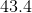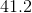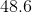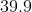Explanation:

Recall the equation that gives the relationship between change in temperature and amount of heat: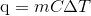where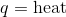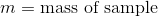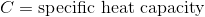, and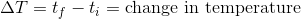Since the question asks for the final temperature, re-arrange the equation to solve for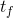.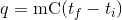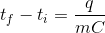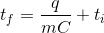Substitute in the given values to solve for the final temperature.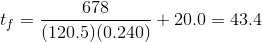Example Question #33 : College Chemistry

The specific heat capacity is defined as the amount of heat energy necessary to change a given amount of a substance by a certain temperature. Which of the following correctly expresses the units of specific heat capacity?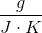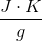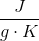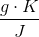Explanation:

For this question, we're given a definition for the specific heat capacity of a substance and we're asked to identify the correct units for this term.

We can also recall the equation that relates all of these terms.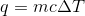Rearranging this expression to isolate the term for the specific heat capacity gives us the following.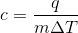Next, we can recall what units would be appropriate to use for each of the following terms in the above expression. The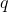term represents heat energy added to or removed from the system, so this value would be in units of joules. Next, theterm represents the mass of the substance, so grams can be used for this term. Finally, the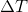term represents the change in temperature of the substance, so we can use the absolute temperature in kelvins here.

Putting all this together gives us the following.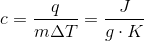Example Question #1 : Thermochemistry And Changes Of State

How much heat is needed to raise the temperature of 10.0 g of water from 10.0 oC to 35.0 oC?

Specific heat capacity for water is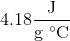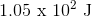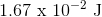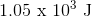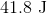Explanation:

Recall the relationship between heat and specific heat capacity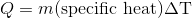Plug in known values and solve for Q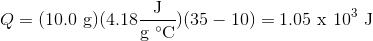Example Question #1 : Solutions, States Of Matter, And Thermochemistry

An insulated container is filled with 50.0 g of water at 15.0 oC. 120.0 g of lead is heated to 100 oC and added to the insulated container. What is the final temperature of the system once it comes to equilibrium?

Specific heat of water isSpecific heat of lead is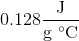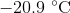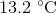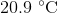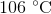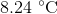Explanation:

Since Pb starts at a higher temperature than the water, we know that energy (in the form of heat) will be transferred from Pb to water. Due to the law of conservation of energy, the exact same amount of energy lost by Pb must be gained by water.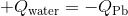Recall thatCombining the two equations, we have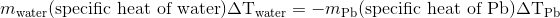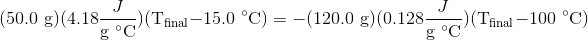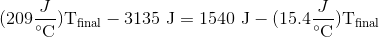Combine like terms then solve for final temperature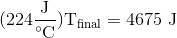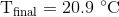Example Question #36 : College Chemistry

How much heat does it require to make a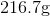block of lead initially at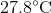go to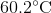? The specific heat capacity of lead is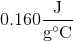.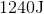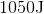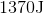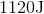Explanation:

Recall the equation that gives the relationship between the change in temperature and the amount of heat: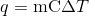, where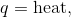,, and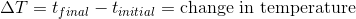Substitute in the given values to find how much heat is required to increase the temperature of the block of lead the specified amount.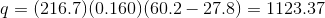Make sure to round the answer to three significant figures.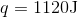All College Chemistry Resources